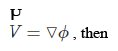# ISRO Scientist or Engineer Mechanical 2007

Instructions

For the following questions answer them individually

Question 11

# If the governing equation fora flow field is given by $$\triangledown^2\phi = 0$$ and the velocity is given byQuestion 12

# With rise in temperature thermal conductivity of airQuestion 13

# In a reversible adiabatic process, the ratio of $$\frac{T_1}{T_2}$$ is equal toQuestion 14

# Chances of occurrenceof cavitation are high if theQuestion 15

# The general equation of continuity for three-dimensional flow of a incompressible fluid for steady flow isQuestion 16

# A liquid compressedin cylinder has a volume of 0.04 m$$^3$$ at 50 kg/cm$$^2$$ and a volume of 0.039 m$$^3$$ at 150 kg/cm$$^2$$. The bulk modulus of liquid isQuestion 17

# The buoyancy depends onQuestion 18

# A fluid jet discharging from a 100 mm diameter orifice has a diameter of 80 mmat its vena contracta. The coefficient of contraction isQuestion 19

# If a jet of water of area ‘A’ strikes with velocity ‘V’ a series of flat plates mounted on a wheel of mean diameter D rotating at N rpm, then force exerted will be equal toQuestion 20

# The maximum height of a siphon for a fluid of specific gravity $$\rho$$ under atmospheric conditions isOR